Courses

# Parsing MCQ - 1

## 20 Questions MCQ Test RRB JE for Computer Science Engineering | Parsing MCQ - 1

Description
This mock test of Parsing MCQ - 1 for Computer Science Engineering (CSE) helps you for every Computer Science Engineering (CSE) entrance exam. This contains 20 Multiple Choice Questions for Computer Science Engineering (CSE) Parsing MCQ - 1 (mcq) to study with solutions a complete question bank. The solved questions answers in this Parsing MCQ - 1 quiz give you a good mix of easy questions and tough questions. Computer Science Engineering (CSE) students definitely take this Parsing MCQ - 1 exercise for a better result in the exam. You can find other Parsing MCQ - 1 extra questions, long questions & short questions for Computer Science Engineering (CSE) on EduRev as well by searching above.
QUESTION: 1

Solution:
QUESTION: 2

### Consider the following two sets of LR(1) items of an LR(1) grammar. X -> c.X, c/d X -> .cX, c/d X -> .d, c/d X -> c.X, \$ X -> .cX, \$ X -> .d, \$ Q. Which of the following statements related to merging of the two sets in the corresponding LALR parser is/are FALSE? 1. Cannot be merged since look aheads are different. 2. Can be merged but will result in S-R conflict. 3. Can be merged but will result in R-R conflict. 4. Cannot be merged since goto on c will lead to two different sets.

Solution:

The given two LR(1) set of items are :
X -> c.X, c/d
X -> .cX, c/d
X -> .d, c/d
and
X -> c.X, \$
X -> .cX, \$
X -> .d, \$

The symbols/terminals after the comma are Look-Ahead symbols. These are the sets of LR(1) (LR(1) is also called CLR(1)) items. The LALR(1) parser combines those set of LR(1) items which are identical with respect to their 1st component but different with respect to their 2nd component. In a production rule of a LR(1) set of items, (A -> B , c) , A->B is the 1st component , and the Look-Ahead set of symbols, which is c here, is the second component. Now we can see that in the sets given, 1st component of the corresponding production rule is identical in both sets, and they only differ in 2nd component (i.e. their look-ahead symbols) hence we can combine these sets to make a a single set which would be :

X -> c.X, c/d/\$
X -> .cX, c/d/\$
X -> .d, c/d/\$

This is done to reduce the total number of parser states. Now we can check the statements given. Statement 1 : The statement is false, as merging has been done because 2nd components i.e. look-ahead were different. Statement 2 : In the merged set, we can't see any Shift-Reduce conflict ( because no reduction even possible, reduction would be possible when a production of form P -> q. is present) Statement 3 : In the merged set, we can't see any Reduce-Reduce conflict ( same reason as above, no reduction even possible, so no chances of R-R conflict ) Statement 4: This statement is also wrong, because goto is carried on Non-Terminals symbols, not on terminal symbols, and c is a terminal symbol. Thus, all statements are wrong, hence option D.

QUESTION: 3

### For the grammar below, a partial LL(1) parsing table is also presented along with the grammar. Entries that need to be filled are indicated as E1, E2, and E3. ∈ is the empty string, \$ indicates end of input, and, | separates alternate right hand sides of productions.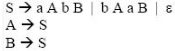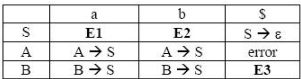Solution:

First(X) - It is the set of terminals that begin the strings derivable from X.
Follow(X) - It is the set of terminals that can appear immediately to the right of X in some sentential form.

Now in the above question,

FIRST(S) = {a, b, epsilon} FIRST(A) = FIRST(S) = {a, b, epsilon} FIRST(B) = FIRST(S) = {a, b, epsilon}
FOLLOW (A) = {b , a}
FOLLOW (S) = {\$} U FOLLOW (A) = {b , a , \$}
FOLLOW (B) = FOLLOW (S) = {b ,a , \$}

epsilon corresponds to empty string.

QUESTION: 4

For the grammar below, a partial LL(1) parsing table is also presented along with the grammar. Entries that need to be filled are indicated as E1, E2, and E3. ∈ is the empty string, \$ indicates end of input, and, | separates alternate right hand sides of productions.Q.

The appropriate entries for E1, E2, and E3 are

Solution:

As we need to find entries E1, E2 and E3 which are against Non terminals S and B, so we will deal with only those productions which have S and B on LHS. Here representing the parsing table as M[X , Y], where X represents rows( Non terminals) and Y represents columns(terminals).

Rule 1: if P --> Q is a production, for each terminal 't' in FIRST(Q) add P-->Q to M [ P , t ]
Rule 2 : if epsilon is in FIRST(Q), add P --> Q to M [P , f] for each f in FOLLOW(P).

For the production rule S--> aAbB, it will be added to parsing table at the position M[ S , FIRST(aAbB)], Now FIRST(aAbB) = {a}, hence add S--> aAbB to M[S , a] which is E1. For the production rule S--> bAaB, it will be added to parsing table at the position M[ S , FIRST(bAaB)], Now FIRST(bAaB) = {b}, hence add S--> bAaB to M[ S , b] which is E2 For the production rule S--> epsilon , it will be added to parsing table at the position M[ S , FOLLOW(S)], Now FOLLOW(S) = {a , b , \$}, hence add S --> epsilon to M[ S , a] and M[ S , b] which are again E1 and E2 respectively. For the production rule B --> S, it will be added to parsing table at the position M[ B , FIRST(S)], Now FIRST(S) also contains epsilon, hence add B --> S to M[ S , FOLLOW(B)], and FOLLOW(B) contains {\$}, i.e. M[S , \$] which is E3. epsilon corresponds to empty string.

QUESTION: 5

The grammar S → aSa | bS | c is

Solution:

First(aSa) = a
First(bS) = b
First(c) = c
All are mutually disjoint i.e no common terminal between them, the given grammar is LL(1).
As the grammar is LL(1) so it will also be LR(1) as LR parsers are more powerful then LL(1) parsers. and all LL(1) grammar are also LR(1) So option C is correct.
Below are more details. A grammar is LL(1) if it is possible to choose the next production by looking at only the next token in the input string.
Formally, grammar G is LL(1) if and only if
For all productions A → α1 | α2 | ... | αn,
First(αi) ∩ First(αj) = ∅, 1 ≤ i,j ≤ n, i ≠ j.
For every non-terminal A such that First(A) contains ε,
First(A) ∩ Follow(A) = ∅

QUESTION: 6

Match all items in Group 1 with correct options from those given in Group 2.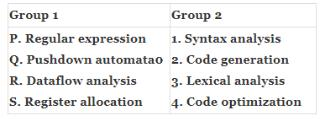Solution:

Regular expressions are used in syntax analysis. Pushdown automata is related to context free grammar which is related to syntax analysis. Dataflow analysis is done in code optimization. Register allocation is done in code generation.

QUESTION: 7

Which of the following statements are TRUE?

I. There exist parsing algorithms for some programming languages whose complexities are less than O(n3).
II. A programming language which allows recursion can be implemented with static storage allocation.
III. No L-attributed definition can be evaluated in The framework of bottom-up parsing.
IV. Code improving transformations can be performed at both source language and intermediate code level.

Solution:

II is false, in recursion, compiler cannot determine the space needed for recursive calls. III is false.

QUESTION: 8

Which of the following describes a handle (as applicable to LR-parsing) appropriately?

Solution:

Let's first understand the terminology used here. LR Parsing - Here 'L' stands for Left to Right screening of input string, and 'R' stands for Right Most Derivation in Reverse (because it is about bottom-up parsing). Sentential Form - Suppose For a given Context Free Grammar G, we have a start symbol S, then to define Language generated by Grammar G, i.e. L(G), we start the derivation starting from S using the production rules of the grammar. After one complete derivation we get a string w which consists of only terminal symbols, i.e. w belongs to L(G). Then we can say that w is a sentence of the Grammar G. Now, while the derivation process, if it gets some form q, where q may contain some Non-terminal then we say that q is a sentential form of the Grammar G. Even the start symbol S is also the sentential form of the Grammar G (because it also contains Non-terminal S).

For Ex :
Grammar is :
S-> aAcBe
A->Ab|b
B->d
Input string : abbcde

Derivation : (Top-Down, Right Most Derivation)
S->aAcBe
->aAcde
->aAbcde
->abbcde

Here {abbcde} is the sentence of the Grammar(because it contains only terminal symbols), and {S, aAcBe, aAcde, aAbcde} are the sentential forms of the G (because these forms contain non-terminals during the derivation process) Now, let's look at the question. The question is related to LR parsing which is a bottom-up parsing. Let's take the same grammar above, as it is a bottom up parsing we need to start from the string "abbcde" and try to get S using production rules.

: abbcde
->aAbcde (using A-> b)
->aAcde (using A-> Ab)
->aAcBe (using B -> d)
->S (using S-> aAcBe)

The above process is called reduction. The RHS of the Production which is being replaced by their LHS is called Handle, So , { b, Ab, d, aAcBe} are handles, and replacing it with its LHS is called Handle-Pruning. Hence option D suits best.

QUESTION: 9

An LALR(1) parser for a grammar G can have shift-reduce (S-R) conflicts if and only if

Solution:

Both LALR(1) and LR(1) parser uses LR(1) set of items to form their parsing tables. And LALR(1) states can be find by merging LR(1) states of LR(1) parser that have the same set of first components of their items. i.e. if LR(1) parser has 2 states I and J with items A->a.bP,x and A->a.bP,y respectively, where x and y are look ahead symbols, then as these items are same with respect to their first component, they can be merged together and form one single state, let's say K. Here we have to take union of look ahead symbols. After merging, State K will have one single item as A->a.bP,x,y . This way LALR(1) states are formed (i.e. after merging the states of LR(1)). Now, S-R conflict in LR(1) items can be there whenever a state has items of the form :
A-> a.bB , p
C-> d. , b
i.e. it is getting both shift and reduce at symbol b, hence a conflict.
Now, as LALR(1) have items similar to LR(1) in terms of their first component, shift-reduce form will only take place if it is already there in LR(1) states. If there is no S-R conflict in LR(1) state it will never be reflected in the LALR(1) state obtained by combining LR(1) states. Note: But this process of merging may introduce R-R conflict, and then the Grammar won't be LALR(1).

QUESTION: 10

Which one of the following is a top-down parser?

Solution:

Recursive Descent parsing is LL(1) parsing which is top down parsing.

QUESTION: 11

Consider the grammar with non-terminals N = {S,C,S1},terminals T={a,b,i,t,e}, with S as the start symbol, and the following set of rules:

S --> iCtSS1|a
S1 --> eS|ϵ
C --> b

The grammar is NOT LL(1) because:

Solution:

A  LL(1) grammar doesn't give to multiple entries in a single cell of its parsing table. It has only single entry in a single cell, hence it should be unambiguous.

Option A is wrong. Grammar is not left recursive. For a grammar to be left recursive a production should be of form A->Ab, where A is a single Non-Terminal and b is any string of grammar symbols.

Option B is wrong. Because a right recursive grammar has nothing to do with LL(1).

Option D is wrong. Because the given grammar is clearly a Context Free Grammar. A grammar is CFG if it has productions of the form A->(V∪T)* , where A is a single non-terminal and V is a set of Non-terminals and T is a set of Terminals.

Hence Option C should be the correct one. i.e. the grammar is ambiguous.

But let's see how the grammar is ambiguous.

If the grammar is ambiguous then it should give multiple entry in a cell while making its parsing table. And Parse table is made with the aid of two functions : FIRST and FOLLOW.

A parsing table of a grammar will not have multiple entries in a cell( i.e. will be a LL(1) grammar) if and only if the following conditions hold for each production of the form A->α|β 1) FIRST(α)  ∩ FIRST(β) =  Φ2) if FIRST(α) contains ' ε ' then FIRST(α) ∩ FOLLOW (A) =  Φ and vice-versa.

Now,

• For the production , S->iCtSS1|a, rule 1 is satisfied, because FIRST(iCtSS1) ∩ FIRST(a) = {i} ∩ {a} = Φ
• For the production, S1->eS|ε, rule 1 is satisfied, as FIRST(eS) ∩ FIRST(ε) = {e} ∩ {ε} = Φ. But here due to 'ε' in FIRST, we have to check for rule 2. FIRST(eS)  ∩ FOLLOW(S1) = {e} ∩ {e, \$} ≠ Φ. Hence rule 2 fails in this production rule. Therefore there will be multiple entries in the parsing table, hence the grammar is ambiguous and not LL(1).
QUESTION: 12

Consider the following two statements:

P: Every regular grammar is LL(1)
Q: Every regular set has a LR(1) grammar

Q. Which of the following is TRUE?

Solution:

A regular grammar can also be ambiguous also For example, consider the following grammar,
S → aA/a
A → aA/ε
In above grammar, string 'a' has two leftmost derivations.

(1) S → aA
(2)   S → a
S->a (using A->ε)

And LL(1) parses only unambiguous grammar, so statement P is False. Statement Q is true is for every regular set, we can have a regular grammar which is unambiguous so it can be parse by LR parser.
So option C is correct choice

QUESTION: 13

Consider the following grammar.

S -> S * E
S -> E
E -> F + E
E -> F
F -> id

Consider the following LR(0) items corresponding to the grammar above.

(i) S -> S * .E
(ii) E -> F. + E
(iii) E -> F + .E

Q. Given the items above, which two of them will appear in the same set in the canonical sets-of-items for the grammar?

Solution:

Let's make the LR(0) set of items. First we need to augment the grammar with the production rule S' -> .S , then we need to find closure of items in a set to complete a set. Below are the LR(0) sets of items.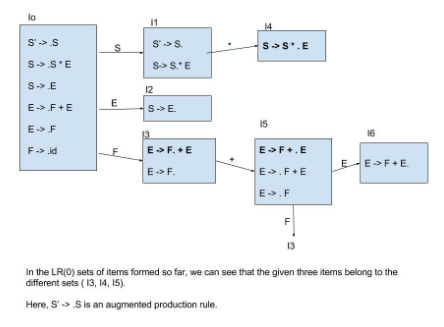QUESTION: 14

A canonical set of items is given below

S --> L. > R
Q --> R.

On input symbol < the set has

Solution:

The question is asked with respect to the symbol  ' < ' which is not present in the given canonical set of items. Hence it is neither a shift-reduce conflict nor a reduce-reduce conflict on symbol '<'. Hence D is the correct option. But if the question would have asked with respect to the symbol  ' > ' then it would have been a shift-reduce conflict.

QUESTION: 15

Consider the grammar defined by the following production rules, with two operators ∗ and +

S --> T * P
T --> U | T * U
P --> Q + P | Q
Q --> Id
U --> Id

Q. Which one of the following is TRUE?

Solution:

From the grammar we can find out associative by looking at grammar.

Let us consider the 2nd production T -> T * U
T is generating T*U recursively (left recursive) so * is left associative.
Similarly
P -> Q + P
Right recursion so + is right associative. So option B is correct.

NOTE: Above is the shortcut trick that can be observed after drawing few parse trees. One can also find out correct answer by drawing the parse tree.

QUESTION: 16

Consider the following grammar:

S → FR
R → S | ε
F → id

In the predictive parser table, M, of the grammar the entries M[S, id] and M[R, \$] respectively.

Solution:

Here representing the parsing table as M[X , Y], where X represents rows(Non terminals) and Y represents columns(terminals). Here are the rules to fill the parsing table. For each distinct production rule A->α, of the grammar, we need to apply the given rules:
Rule 1: if A --> α is a production, for each terminal 'a' in FIRST(α), add A-->α to M[ A , a]
Rule 2 : if ' ε ' is in FIRST(α), add A --> α to M [A , b] for each 'b' in FOLLOW(A). As Entries have been asked corresponding to Non-Terminal S and R, hence we only need to consider their productions to get the answer. For S → FR, according to rule 1, this production rule should be placed at the entry M[ S, FIRST(FR)], and from the given grammar, FIRST(FR) ={id}, hence S->FR is placed in the parsing table at entrM[S , id]. Similarly, For R → S, this production rule should be placed at entry M[ R, FIRST(S)], and as FIRST(S) = FIRST(F) = {id} hence, R->S is placed at entry M[R,id] and For R->ε, as FIRST(ε) = {ε}, hence rule 2 should be applied, therefore, this production rule should be placed in the parsing table at entry M[R,FOLLOW(R)], and FOLLOW(R) = FOLLOW(S) = {\$}, hence R->ε is placed at entry M[R, \$]. Therefore Answer is option A. Visit the Following links to Learn how to find First and Follow sets.

QUESTION: 17

Consider the following translation scheme. S → ER R → *E{print("*");}R | ε E → F + E {print("+");} | F F → (S) | id {print(id.value);} Here id is a token that represents an integer and id.value represents the corresponding integer value. For an input '2 * 3 + 4', this translation scheme prints

Solution:

Background Required to solve the question - Syntax Directed Translation and Parse Tree Construction.

xplanation : We are given L-Attributed Syntax Directed Translation as semantic actions like printf statements are inserted anywhere on the RHS of production (R → *E{print(“*”);}R). After constructing the parse tree as shown below from the given grammar, we will follow depth first order left to right evaluation in order to generate the final output.

Parse Tree: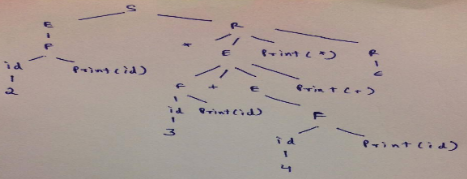Just follow the arrows in the picture (This is actually Depth first left to right evaluation) and the moment we take exit from any child which is printf statement in this question, we print that symbol which can be a integer value or ‘*’ or ‘+’.

Evaluation :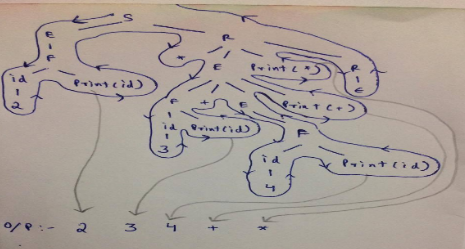QUESTION: 18

The grammar A → AA | (A) | ε is not suitable for predictive-parsing because the grammar is

Solution:

nswer (B) is correct because grammar is left recursive, hence the parser may fall into a loop. Answer A is not correct because ambiguity can occur from both left or right recursion.

QUESTION: 19

Consider the grammar

E → E + n | E × n | n

For a sentence n + n × n, the handles in the right-sentential form of the reduction are

Solution:

E → E + n            {Applying E → E + n}
→ E + E * n      {Applying E → E * n}
→ E + n * n       {Applying E → n}
→ n + n * n        {Applying E → n}

QUESTION: 20

Consider the grammar

S → (S) | a

Let the number of states in SLR(1), LR(1) and LALR(1) parsers for the grammar be n1, n2 and n3 respectively. The following relationship holds good

Solution:

LALR(1) is formed by merging states of LR(1) (also called CLR(1)), hence no of states in LALR(1) is less than no of states in LR(1), therefore n3 < n2. And SLR(1) and LALR(1) have same no of states, i.e (n1 = n3).

Hence n1 = n3 < n2